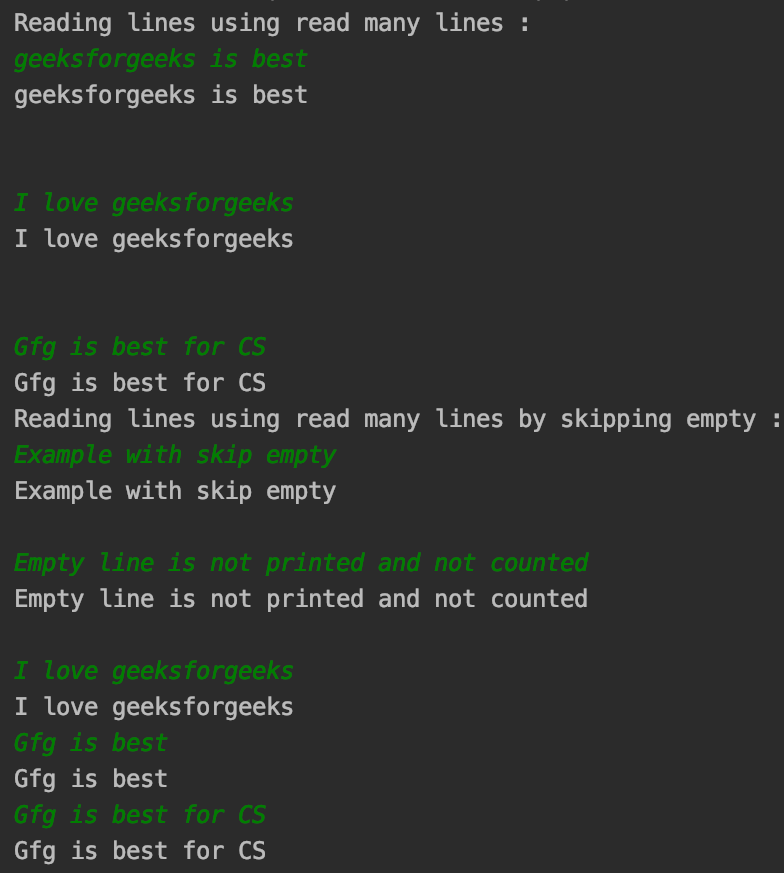# easyinput module in Python

• Last Updated : 29 Jul, 2021

easyinput module in Python offers an easy input interface analogous to cin stream in C++. It supports multiple data types including files and provides functionalities such as input till different data types, multi-line input, etc.

### Installation

To install this module type the below command in the terminal.

`pip install easyinput`

### Function Used

• read(*type, amount=1, file, as_list): Returns tokens from input stream.

Parameters:

• type : List of data types, for each type corresponding token is returned.
• amount : For repetition of given type for specific number of times.
• as_list : If true, stream of tokens is returned as list, otherwise, generator is returned.
• file : File entity stream.
• read_many(*types, amount=1, file=_StdIn): Reads token from input stream unless the element of other type is entered.

Example 1: Working of read() and read_many()

## Python3

 `from` `easyinput ``import` `read_many, read`  `a ``=` `read(``int``)``b ``=` `read(``str``)` `print``(``"The elements using read : "``)``print``(a, b)` `print``(``"Integer inputs using read many : "``)``for` `num ``in` `read_many(``int``):``    ``print``(num)` `# reading the string after integers``print``(read())`

Output :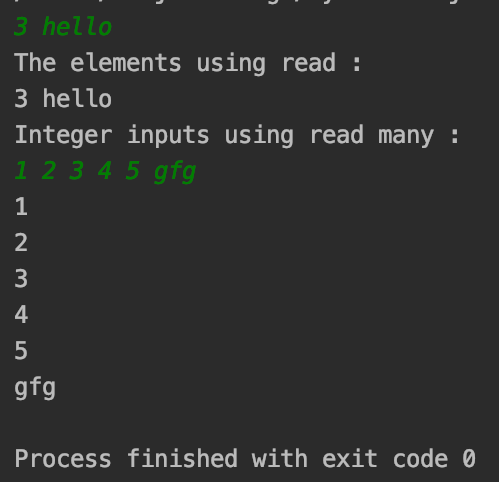Example 2: Using amount() and as_list()

Usually, read() returns a list when working with multiple arguments in read(). If we need a generator to be used, not a list, as_list() can be set to false. The advantage it can have is that it may avoid iteration of the list to access and populate to different data types.

## Python3

 `from` `easyinput ``import` `read`  `# input int, str, int chain 3 times``multi_input ``=` `read(``int``, ``str``, amount``=``2``)` `# printing type and input``print``(``type``(multi_input))``print``(multi_input)` `# putting as_list = False``print``(``"Using as_list false : "``)``multi_input ``=` `read(``int``, ``str``, amount``=``2``, as_list``=``False``)` `print``(``type``(multi_input))``print``(multi_input)`

Output :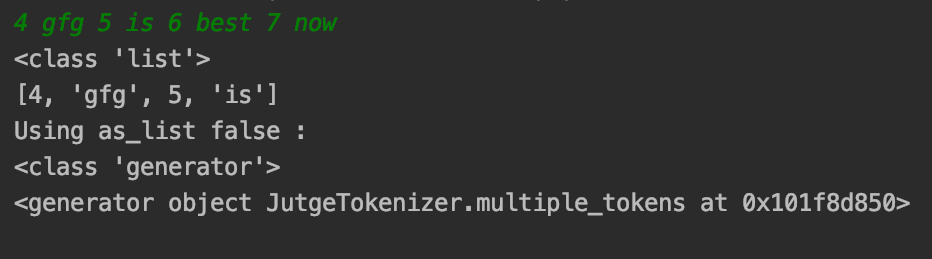Using amount() and as_list()

Example 3: File input using read_many()

The read() and read_many() functions provide the functionality to get files as an input stream to get data from files and render on console, or to any file using file parameter.

Code :

## Python3

 `from` `easyinput ``import` `read_many` `print``(``"Getting integer inputs from files : "``)``with ``open``(``'gfg_file_input'``) as inp_file:``    ``for` `ele ``in` `read_many(``int``, ``file``=``inp_file):``        ``print``(ele)`

Output :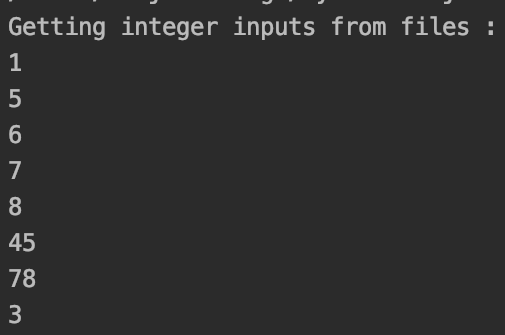Output numbers

Example 4: Using Custom data type with read()

Apart from primitive data types, read() can accept class instances accepting strings as input which can be used to translate to custom types for working. The example below gets lowercase words.

## Python3

 `from` `easyinput ``import` `read`  `class` `ToLower:``    ``def` `__init__(``self``, ele):``        ``self``.ele ``=` `ele.lower()` `    ``def` `print_ele(``self``):``        ``print``(``self``.ele)`  `# Gets object of ToLower class``ele ``=` `read(ToLower)` `# printing the word``print``(``"Lowercase string : "``)``ele.print_ele()`

Output :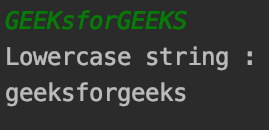Getting Input converting to lowercase

### Working with read_many_lines()

Similar to read_many(), the difference being it reads whole lines at once rather than moving to newline at spaces. Reads the whole line.

Syntax:

Parameters:
rstrip : Skips all the trailing newline characters that are entered. By default value is True.
skip_empty :  Defaults to false, when set to True, skips the lines which are just empty characters.

Code :

## Python3

 `from` `easyinput ``import` `read_many_lines` `print``(``"Reading lines using read many lines : "``)``count ``=` `1``for` `line ``in` `read_many_lines():``    ``print``(line)``    ``count ``=` `count ``+` `1``    ``if` `count > ``5``:``        ``break` `print``(``"Reading lines using read many lines by skipping empty : "``)``count ``=` `1``for` `sline ``in` `read_many_lines(skip_empty``=``True``):``    ``print``(sline)``    ``count ``=` `count ``+` `1``    ``if` `count > ``5``:``        ``break`

Output :